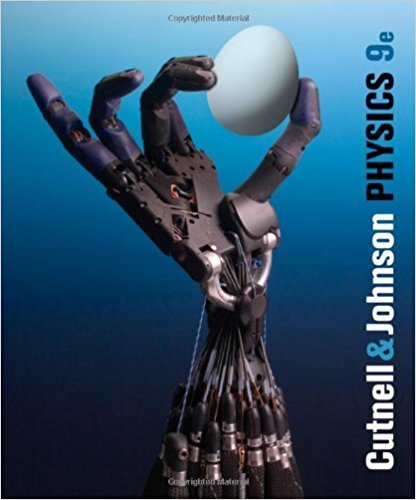×
Log in to StudySoup
Get Full Access to Physics, - 9 Edition - Chapter 23 - Problem 23.4
Join StudySoup for FREE
Get Full Access to Physics, - 9 Edition - Chapter 23 - Problem 23.4

Already have an account? Login here
×
Reset your password

# The drawing shows three ac circuits: one contains aISBN: 9780470879528 211

## Solution for problem 23.4 Chapter 23

Physics, | 9th Edition

• Textbook Solutions
• 2901 Step-by-step solutions solved by professors and subject experts
• Get 24/7 help from StudySoup virtual teaching assistantsPhysics, | 9th Edition

4 5 1 399 Reviews
23
4
Problem 23.4

The drawing shows three ac circuits: one contains a resistor, one a capacitor, and one an inductor. The frequency of each ac generator is reduced to one-half its initial value. Which circuit experiences (a) the greatest increase in current and (b) the least change in current?

Step-by-Step Solution:
Step 1 of 3

Physics 2080 Chapter 16 Temperature and Heat Part B January 12, 2016 Amanda Biddlecome 1) Heat -­‐an object with a higher temperature=hot -­‐heat spontaneously flows always from hot to cold -­‐heat=energy *unit=Joules -­‐Int

Step 2 of 3

Step 3 of 3

##### ISBN: 9780470879528

Since the solution to 23.4 from 23 chapter was answered, more than 345 students have viewed the full step-by-step answer. The answer to “The drawing shows three ac circuits: one contains a resistor, one a capacitor, and one an inductor. The frequency of each ac generator is reduced to one-half its initial value. Which circuit experiences (a) the greatest increase in current and (b) the least change in current?” is broken down into a number of easy to follow steps, and 46 words. Physics, was written by and is associated to the ISBN: 9780470879528. The full step-by-step solution to problem: 23.4 from chapter: 23 was answered by , our top Physics solution expert on 01/04/18, 08:42PM. This full solution covers the following key subjects: . This expansive textbook survival guide covers 32 chapters, and 3930 solutions. This textbook survival guide was created for the textbook: Physics,, edition: 9.

Unlock Textbook Solution

Enter your email below to unlock your verified solution to:

The drawing shows three ac circuits: one contains a